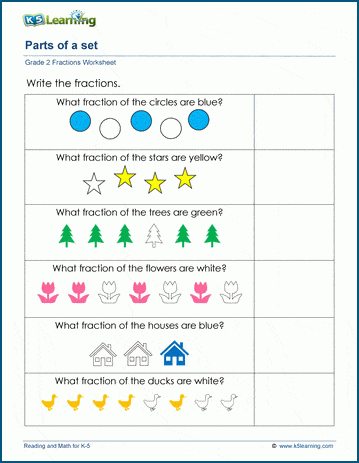# Writing fractions of a set

## Math worksheets: writing parts of a set as fractions

Students are given a set of objects (e.g. 4 stars) and asked a question about the set (e.g. What fraction of the stars are yellow?).  Students learn that the denominator of the fraction represents the total number of objects in the set, and the numerator represents the  number of objects with the chosen property.## More fractions worksheets

Explore all of our fractions worksheets, from dividing shapes into "equal parts" to multiplying and dividing improper fractions and mixed numbers.

## What is K5?

K5 Learning offers reading and math worksheets, workbooks and an online reading and math program for kids in kindergarten to grade 5.  We help your children build good study habits and excel in school.